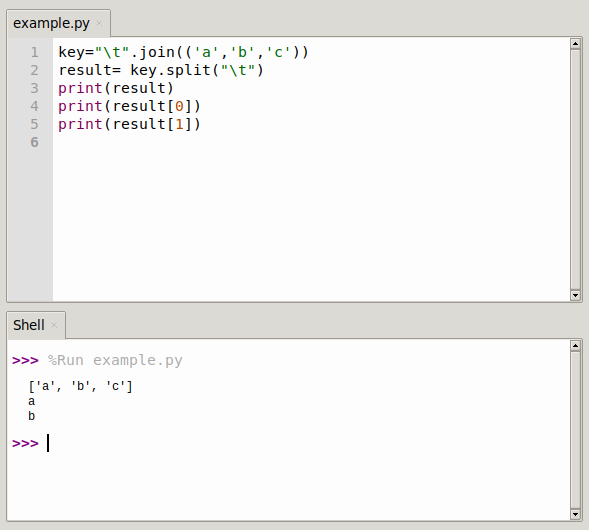# Python string join method

The `join()` function is a string operation function, which also operates on a string. It combines the strings written between the parameters.

``````key="\t".join(('a','b','c'))
result= key.split("\t")
print(result)
print(result)
print(result)``````

The join function connects the specified characters within ().

`",".join ("a", "b", "c")` results in an error because it must be an object in parentheses. If there are more than one, program the tuple, or list.

## Python join() examples

### example 1

Combine a string with different delimiters. The string is split by character and the delimiter is inserted:

``````>>> a="abcd"
>>> ",".join(a)
'a,b,c,d'
>>> "|".join(['a','b','c'])
'a|b|c'
>>> ",".join(('a','b','c'))
'a,b,c'
>>> ",".join({'a':1,'b':2,'c':3})
'a,c,b'``````

## example 2

If the tuple is not used, it will be separated by each character, A, B will also be separated internally.

``````>>> k1="ttt"
>>> k2="ss"
>>> a=k1+k2
>>> ",".join(a)
't,t,t,s,s,s'``````## example 3

You can combine strings using the `+` operator, in fact that works on any sequence like lists or tuples.

``````li = ['hello','python20','!']
str1 = li+' '+ li+' '+li
print(str1)``````

Result:

``hello python20 !``

But Python provides the join() method, which allows the string to be combined. That is a lot shorter to write.

The following is done with `join()`.

``````li = ['hello','python20','!']
print(' '.join(li))``````

Result:

``hello python20 !``